Updating search results...

# 412 Results

View
Selected filters:
• dlc-blended-learning-math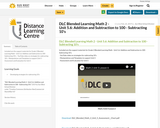Conditional Remix & Share Permitted
CC BY-NC-SA
Rating
0.0 stars

Included are the support materials for Grade 2 Blended Learning Math - Unit 5.6: Addition and Subtraction to 100 - Subtracting 10's:
- YouTube video on strategies for subtracting 10's
- Manipulatives and Templates to support Unit 5
- Assessment and Evaluation for Unit 5

Subject:
Math
Material Type:
Activity/Lab
Assessment
Homework/Assignment
Lesson
Provider:
Sun West Distance Learning Centre (DLC)
02/26/2019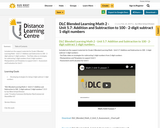Conditional Remix & Share Permitted
CC BY-NC-SA
Rating
0.0 stars

Included are the support materials for Grade 2 Blended Learning Math - Unit 5.7: Addition and Subtraction to 100 - 2-digit subtract 1-digit numbers:
- YouTube video on strategies for subtracting 1-digit numbers from 2-digit numbers
- Manipulatives and Templates to support Unit 5
- Assessment and Evaluation for Unit 5

Subject:
Math
Material Type:
Activity/Lab
Assessment
Homework/Assignment
Lesson
Provider:
Sun West Distance Learning Centre (DLC)
02/26/2019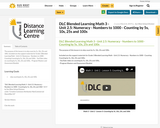Conditional Remix & Share Permitted
CC BY-NC-SA
Rating
0.0 stars

The purpose of this lesson is to skip count by 5s, 10s, 25s and 100s.

Included are the support materials for Grade 3 Blended Learning Math - Unit 2.5: Numeracy - Numbers to 1000 - Counting Counting by 5s, 10s, 25s and 100s:
- YouTube video on counting by 5s, 10s, 25s and 100s,

Subject:
Math
Material Type:
Activity/Lab
Homework/Assignment
Lesson
Provider:
Sun West Distance Learning Centre (DLC)
04/04/2019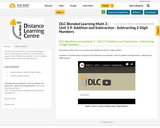Conditional Remix & Share Permitted
CC BY-NC-SA
Rating
0.0 stars

The purpose of this lesson is to use personal strategies to subtract 2-digit numbers.

Included is a YouTube video to support Grade 3 Blended Learning Math - Unit 3.9: Addition and Subtraction - Subtracting 2-Digit Numbers

Subject:
Math
Material Type:
Activity/Lab
Homework/Assignment
Lesson
Provider:
Sun West Distance Learning Centre (DLC)
03/14/2019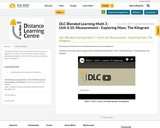Conditional Remix & Share Permitted
CC BY-NC-SA
Rating
0.0 stars

The purpose of this lesson is to estimate and measure mass in kilograms.

Included is a YouTube video to support Grade 3 Blended Learning Math - Unit 4.10: Measurement - Exploring Mass: The Kilogram.

Subject:
Math
Material Type:
Activity/Lab
Homework/Assignment
Lesson
Provider:
Sun West Distance Learning Centre (DLC)
04/04/2019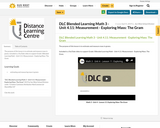Conditional Remix & Share Permitted
CC BY-NC-SA
Rating
0.0 stars

The purpose of this lesson is to estimate and measure mass in grams.

Included is a YouTube video to support Grade 3 Blended Learning Math - Unit 4.11: Measurement - Exploring Mass: The Gram.

Subject:
English Language Arts
Media Studies
Material Type:
Activity/Lab
Homework/Assignment
Lesson
Provider:
Sun West Distance Learning Centre (DLC)
04/04/2019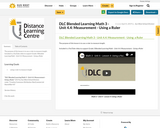Conditional Remix & Share Permitted
CC BY-NC-SA
Rating
0.0 stars

The purpose of this lesson is to use a ruler to measure length.

Included is a YouTube video to support Grade 3 Blended Learning Math - Unit 4.4: Measurement - Using a Ruler

Subject:
Math
Material Type:
Activity/Lab
Homework/Assignment
Lesson
Provider:
Sun West Distance Learning Centre (DLC)
04/04/2019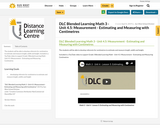Conditional Remix & Share Permitted
CC BY-NC-SA
Rating
0.0 stars

The students will be able to develop referents for centimetres to estimate and measure length, width and height.

Included is a YouTube video to support Grade 3 Blended Learning Math - Unit 4.5: Measurement - Estimating and Measuring Centimetres

Subject:
Math
Material Type:
Activity/Lab
Homework/Assignment
Lesson
Provider:
Sun West Distance Learning Centre (DLC)
04/04/2019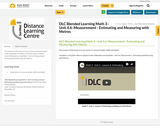Conditional Remix & Share Permitted
CC BY-NC-SA
Rating
0.0 stars

The purpose of this lesson is to use metres to measure length, width and height.

Included is a YouTube video to support Grade 3 Blended Learning Math - Unit 4.6: Measurement - Estimating and Measuring with Metres.

Subject:
Math
Material Type:
Activity/Lab
Homework/Assignment
Lesson
Provider:
Sun West Distance Learning Centre (DLC)
04/04/2019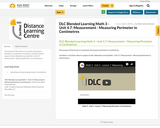Conditional Remix & Share Permitted
CC BY-NC-SA
Rating
0.0 stars

The purpose of this lesson is to estimate and measure perimeter in centimetres.

Included is a YouTube video to support Grade 3 Blended Learning Math - Unit 4.7: Measurement - Measuring Perimeter in Centrimetres.

Subject:
Math
Material Type:
Activity/Lab
Homework/Assignment
Lesson
Provider:
Sun West Distance Learning Centre (DLC)
04/04/2019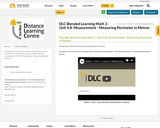Conditional Remix & Share Permitted
CC BY-NC-SA
Rating
0.0 stars

The purpose of this lesson is to measure perimeter in metres.

Included is a YouTube video to support Grade 3 Blended Learning Math - Unit 4.8: Measurement - Measuring Perimeter in Metres.

Subject:
Math
Material Type:
Activity/Lab
Homework/Assignment
Lesson
Provider:
Sun West Distance Learning Centre (DLC)
04/04/2019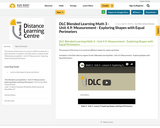Conditional Remix & Share Permitted
CC BY-NC-SA
Rating
0.0 stars

The purpose of this lesson is to construct different shapes for a given perimeter.

Included is a YouTube video to support Grade 3 Blended Learning Math - Unit 4.9: Measurement - Exploring Shapes with Equal Perimeters.

Subject:
Math
Material Type:
Activity/Lab
Homework/Assignment
Lesson
Provider:
Sun West Distance Learning Centre (DLC)
04/04/2019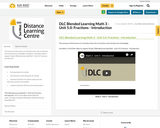Conditional Remix & Share Permitted
CC BY-NC-SA
Rating
0.0 stars

The purpose of this lesson is to introduce the unit on fractions and activate prior knowledge.

Included is a YouTube video to support Grade 3 Blended Learning Math - Unit 5.0: Fractions - Introduction.

Subject:
Math
Material Type:
Activity/Lab
Homework/Assignment
Lesson
Provider:
Sun West Distance Learning Centre (DLC)
04/04/2019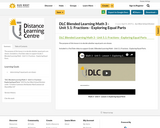Conditional Remix & Share Permitted
CC BY-NC-SA
Rating
0.0 stars

The purpose of this lesson is to decide whether equal parts are shown.

Included is a YouTube video to support Grade 3 Blended Learning Math - Unit 5.1: Fractions - Exploring Equal Parts.

Subject:
Math
Material Type:
Activity/Lab
Homework/Assignment
Lesson
Provider:
Sun West Distance Learning Centre (DLC)
04/04/2019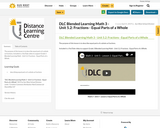Conditional Remix & Share Permitted
CC BY-NC-SA
Rating
0.0 stars

The purpose of this lesson is to describe equal parts of a whole as fractions.

Included is a YouTube video to support Grade 3 Blended Learning Math - Unit 5.2: Fractions - Equal Parts of a Whole.

Subject:
Math
Material Type:
Activity/Lab
Homework/Assignment
Lesson
Provider:
Sun West Distance Learning Centre (DLC)
04/04/2019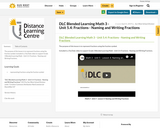Conditional Remix & Share Permitted
CC BY-NC-SA
Rating
0.0 stars

The purpose of this lesson is to represent fractions using the fraction symbol.

Included is a YouTube video to support Grade 3 Blended Learning Math - Unit 5.4: Fractions - Naming and Writing Fractions.

Subject:
Math
Material Type:
Activity/Lab
Homework/Assignment
Lesson
Provider:
Sun West Distance Learning Centre (DLC)
04/04/2019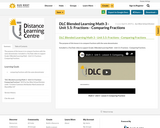Conditional Remix & Share Permitted
CC BY-NC-SA
Rating
0.0 stars

The purpose of this lesson is to compare fractions with the same denominator.

Included is a YouTube video to support Grade 3 Blended Learning Math - Unit 5.5: Fractions - Comparing Fractions.

Subject:
Math
Material Type:
Activity/Lab
Homework/Assignment
Lesson
Provider:
Sun West Distance Learning Centre (DLC)
04/04/2019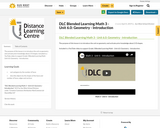Conditional Remix & Share Permitted
CC BY-NC-SA
Rating
0.0 stars

The purpose of this lesson is to introduce the unit on geometry and activate prior knowledge about 2-D shapes.

Included is a YouTube video to support Grade 3 Blended Learning Math - Unit 6.0: Geometry - Introduction.

Subject:
Math
Material Type:
Activity/Lab
Homework/Assignment
Lesson
Provider:
Sun West Distance Learning Centre (DLC)
04/04/2019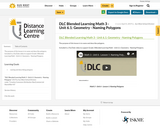Conditional Remix & Share Permitted
CC BY-NC-SA
Rating
0.0 stars

The purpose of this lesson is to name and describe polygons.

Included is a YouTube video to support Grade 3 Blended Learning Math - Unit 6.1: Geometry - Naming Polygons.

Subject:
Math
Material Type:
Activity/Lab
Homework/Assignment
Lesson
Provider:
Sun West Distance Learning Centre (DLC)
04/04/2019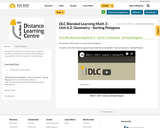Conditional Remix & Share Permitted
CC BY-NC-SA
Rating
0.0 stars

The purpose of this lesson is to name and sort polygons.

Included is a YouTube video to support Grade 3 Blended Learning Math - Unit 6.2: Geometry - Sorting Polygons.

Subject:
Math
Material Type:
Activity/Lab
Homework/Assignment
Lesson
Provider:
Sun West Distance Learning Centre (DLC)